# php设计模式-工厂模式

``````<?php

class Factory
{
//获取一个路由类
public static function getRouteInstance()
{
\$alias = '\Sphp\Core\lib\Route';
\$instance = self::createInstance(\$alias);
return \$instance;
}

//获取一个配置类
public static function getConfInstance()
{
\$alias = '\Sphp\Core\lib\Conf';
\$instance = self::createInstance(\$alias);
return \$instance;
}

//创建一个静态实例
private static function createStaticInstance(\$alias)
{
\$instance = Register::_get(\$alias);
if (!\$instance) {
\$instance = \$alias::getInstance();
Register::_set(\$alias, \$instance);
}
return \$instance;
}

//创建一个实例
private static function createInstance(\$alias)
{
\$instance = Register::_get(\$alias);
if (!\$instance) {
\$instance = new \$alias();
Register::_set(\$alias, \$instance);
}
return \$instance;
}

}
``````

``````\$route = \Sphp\Core\Factory::getRouteInstance();
``````

##### 热门推荐
•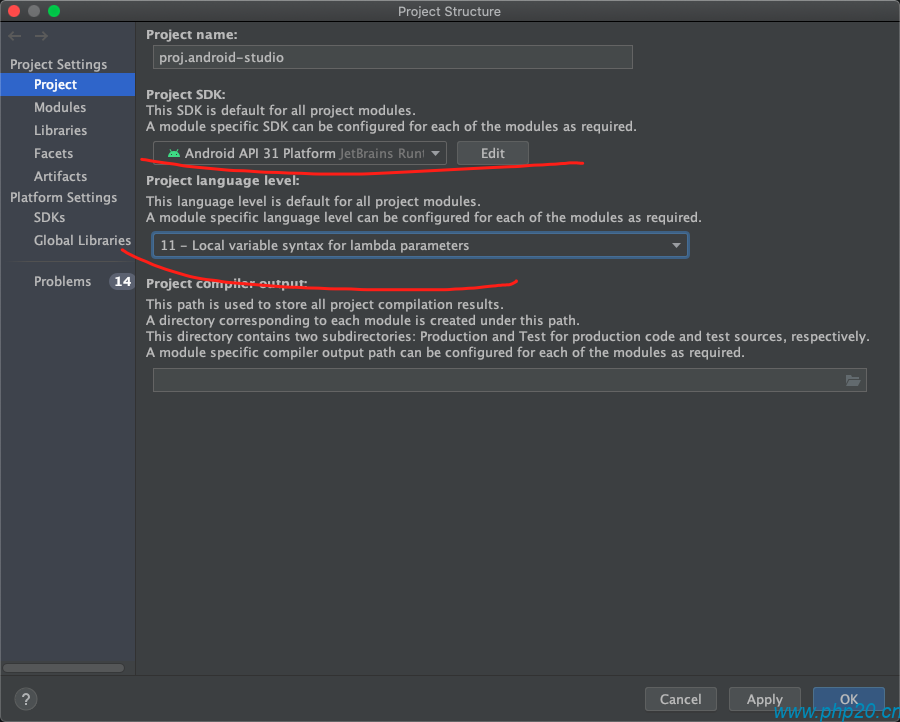浏览(1,986)
•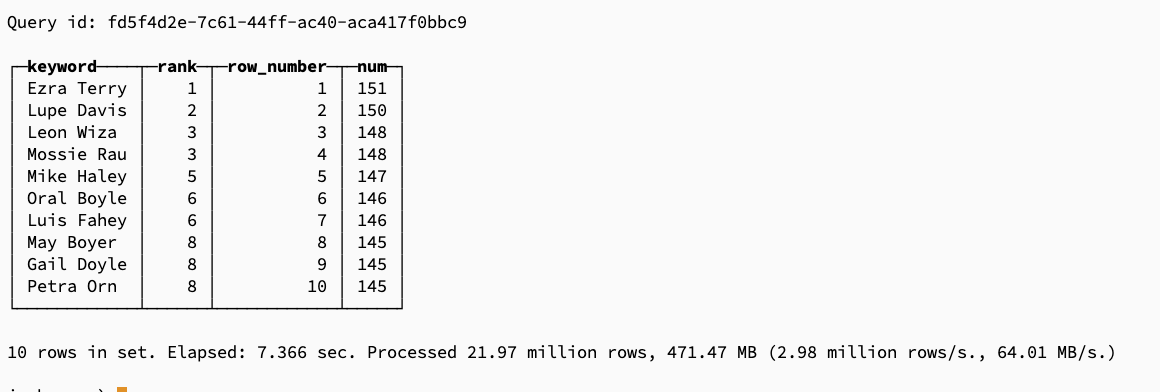浏览(791)
•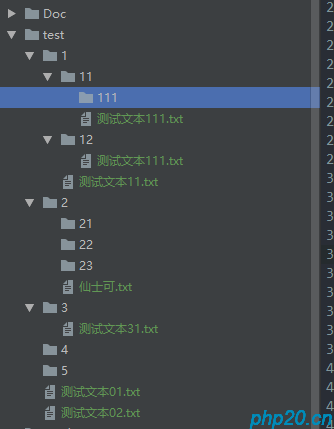浏览(782)
••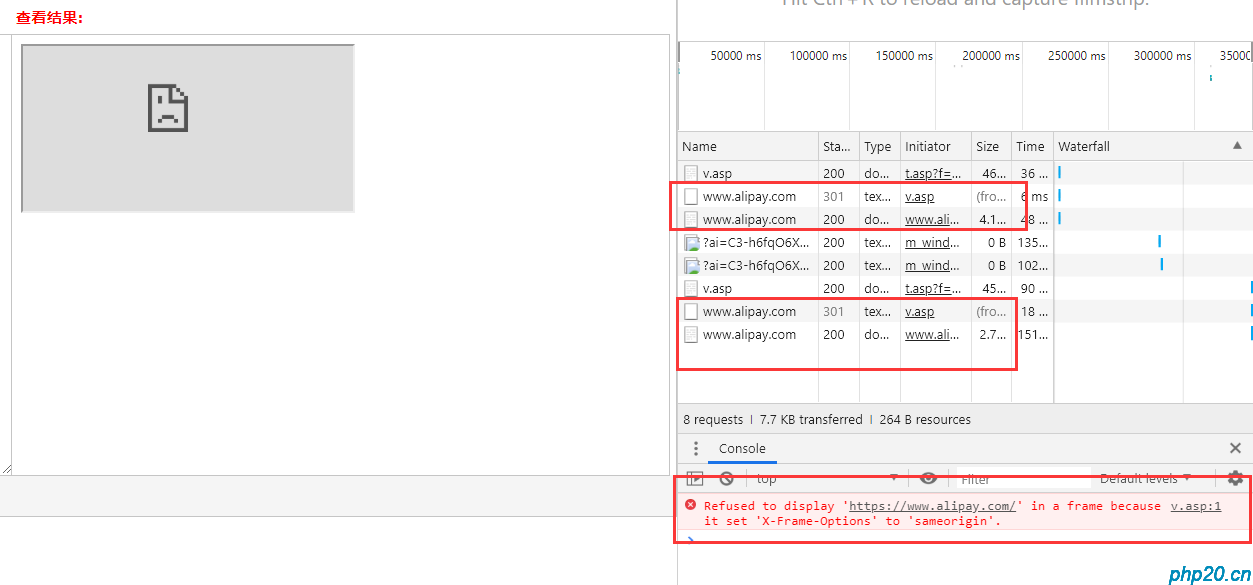浏览(685)
•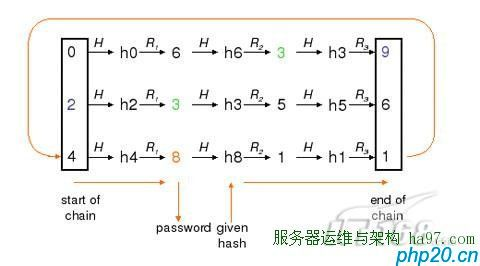•浏览(641)
•浏览(539)
•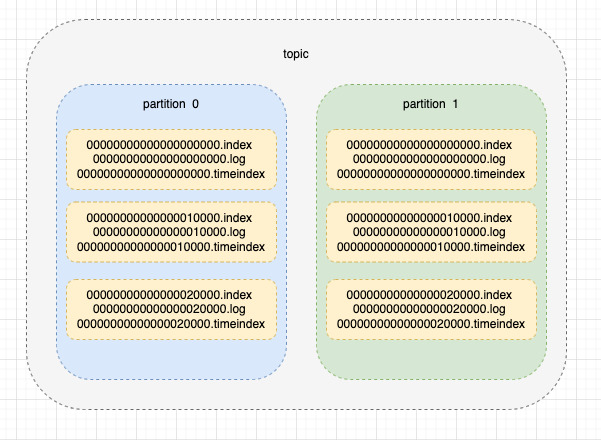浏览(487)
•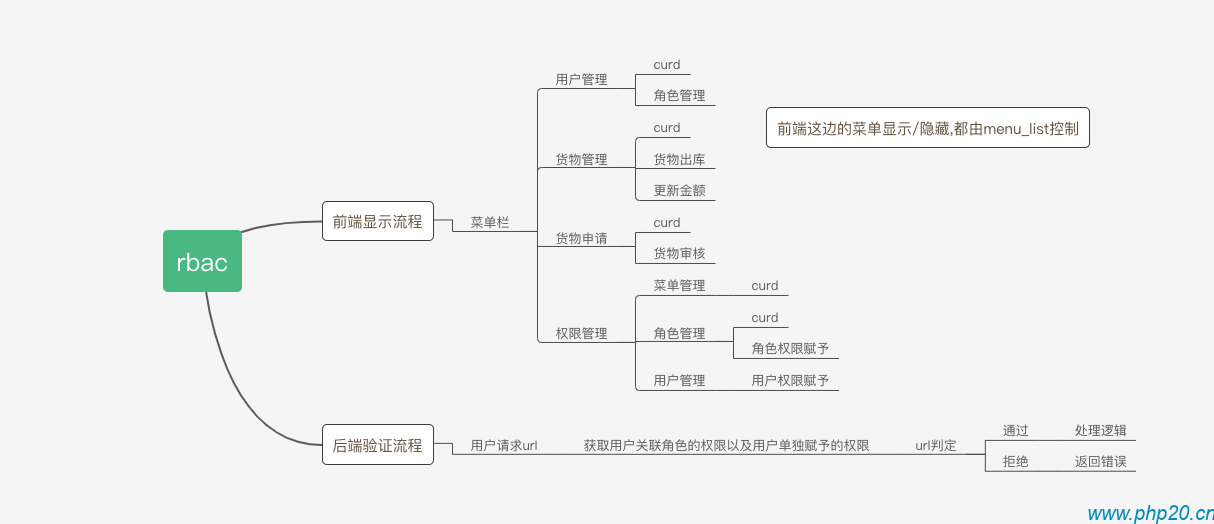浏览(477)